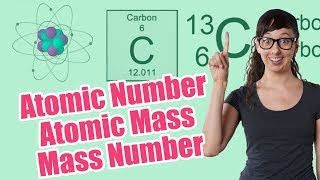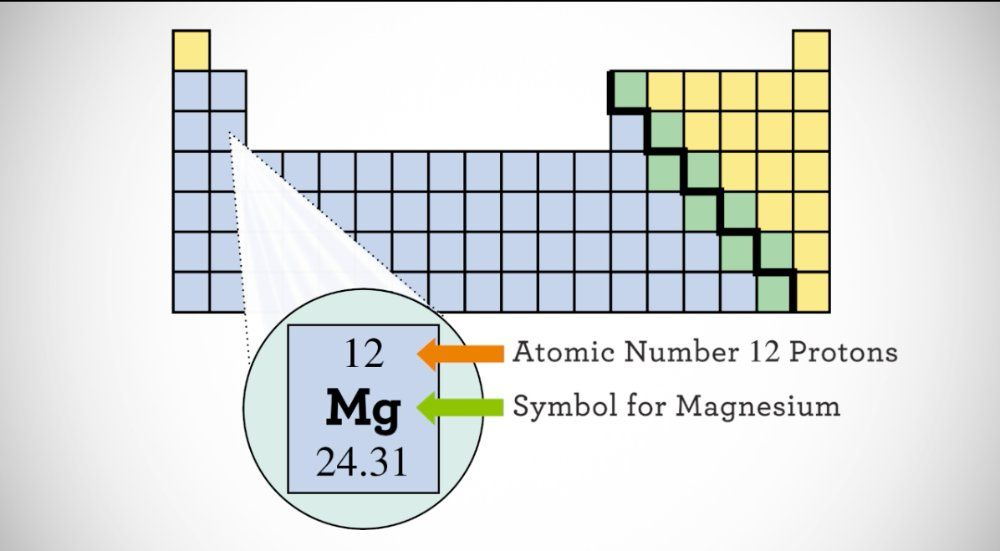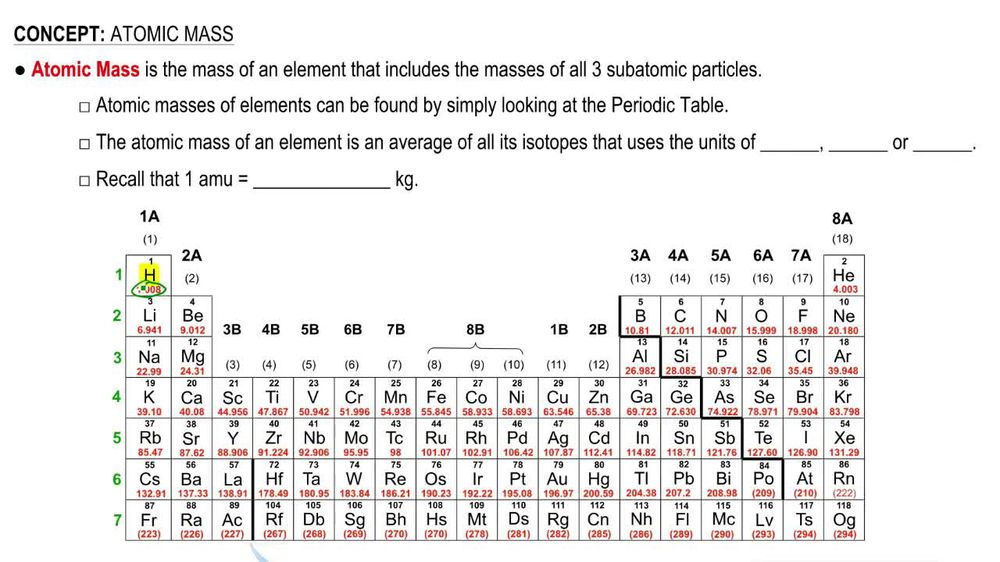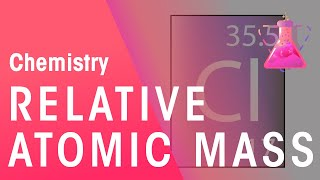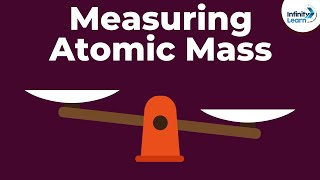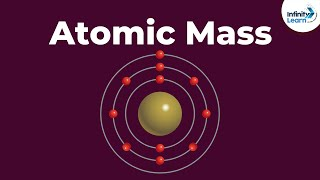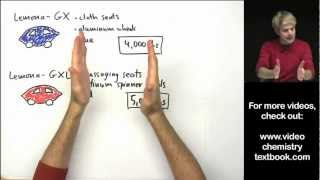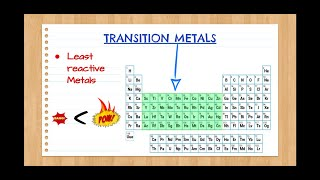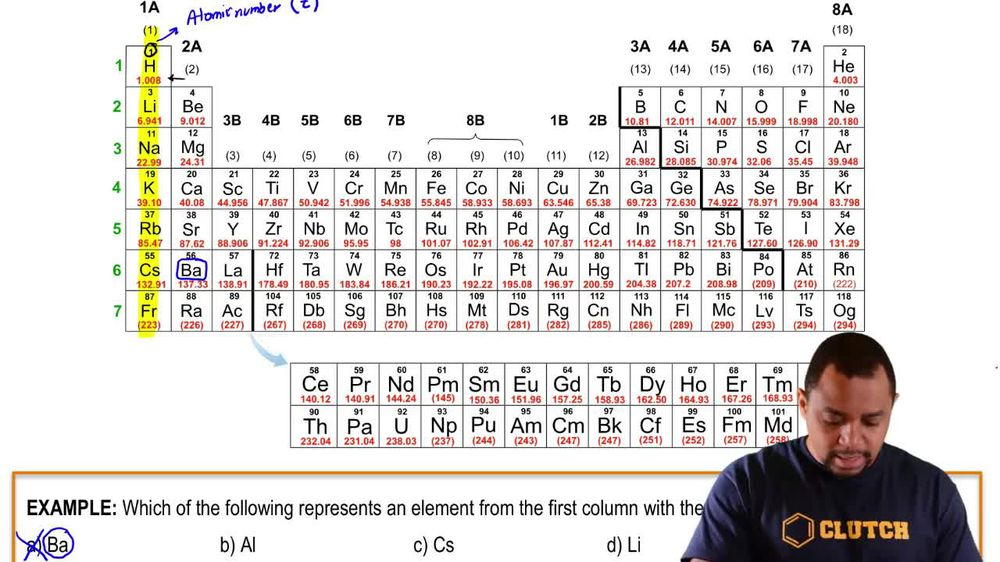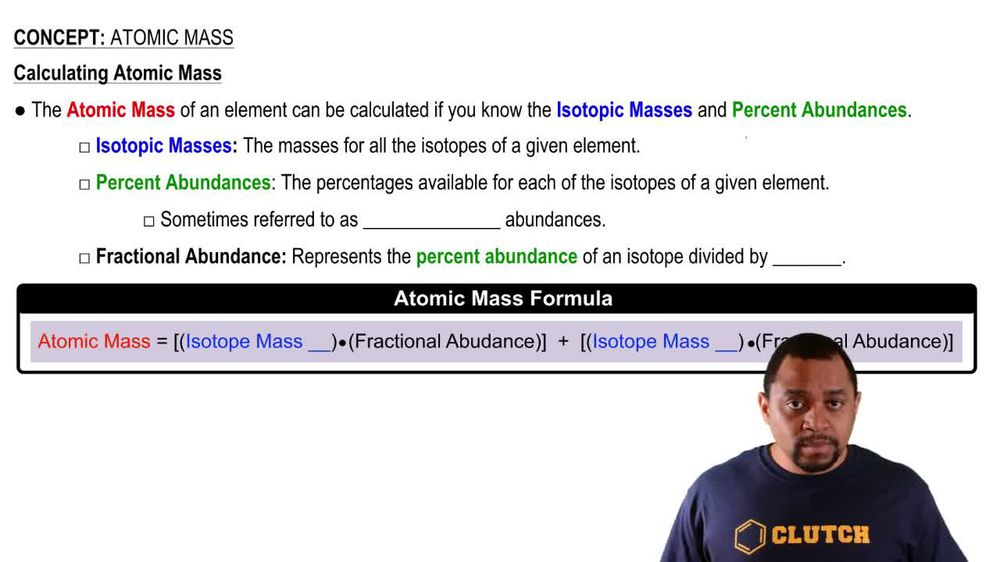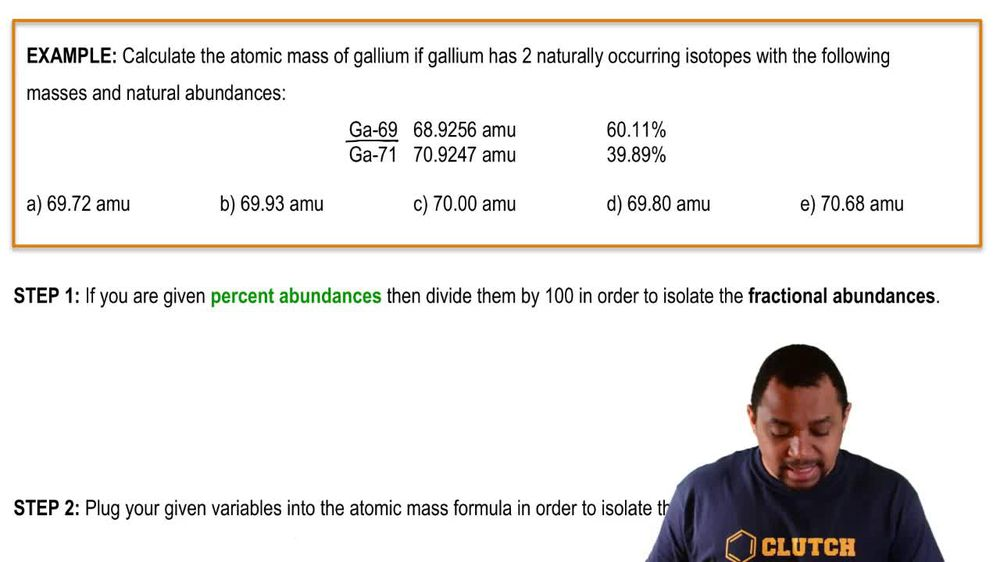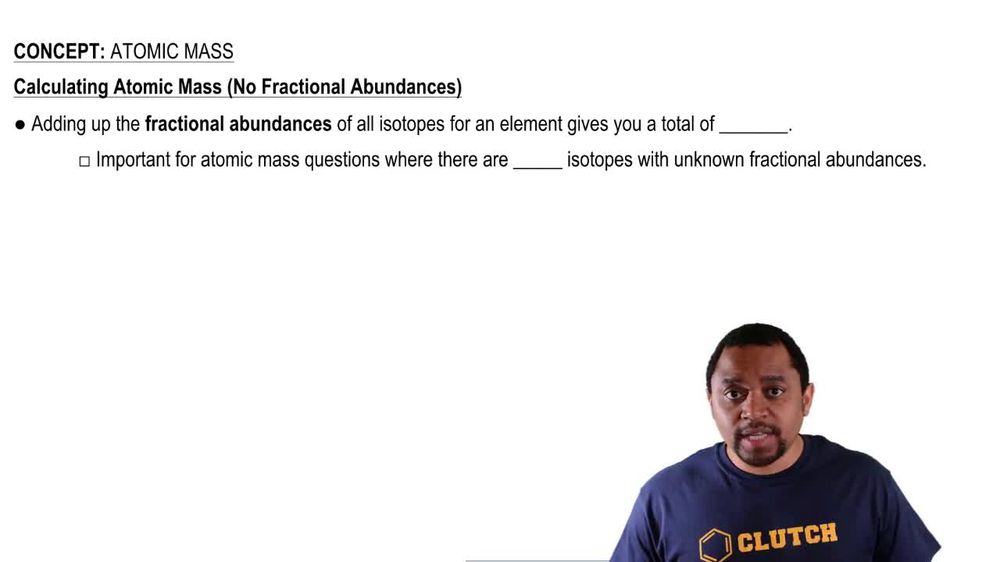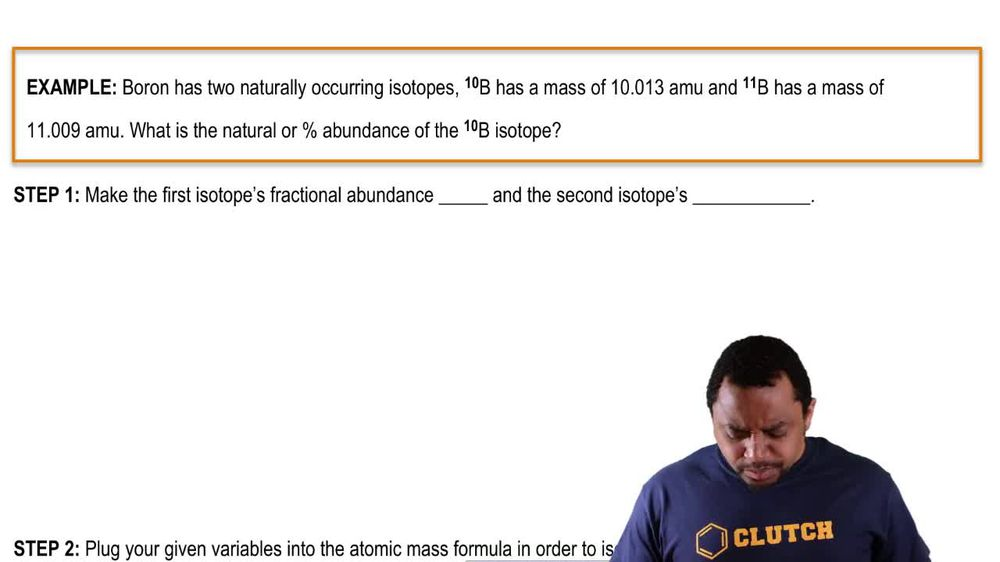Start typing, then use the up and down arrows to select an option from the list.
1. 2. Atoms & Elements2. Atomic Mass
Problem

# Silver is composed of two naturally occurring isotopes: Ag-107 (51.839%) and Ag-109. The ratio of the masses of the two isotopes is 1.0187. What is the mass of Ag-107?

Relevant Solution3m
Play a video:
Hello everyone today. We have the following problem to solve. Copper has two naturally occurring isotopes copper 63 and copper 65. Copper 63 has a natural abundance of 69.15%, calculate the mass of copper 63 if the mass ratio is 1.0318. So the first thing to acknowledge is our formula of finding our atomic mass, which is the atomic mass is equal to the Mass Times The fraction of our isotope one plus the mass times fraction of our isotope too. To find the percentage of copper 65, We take 100 And we subtract by our known percentage, which is copper 63, which is 69.15%. To give us 30.85%. Copper 65. We can then find the fractions of both 63 and 65. So this is our first step. Our second step is to find the fraction. So our fraction of copper 63 Is going to be equal to 0.6915. It's simply taking our percentages and dividing it by 100 and our fraction of our copper 65 is going to be 0. 85 from our periodic table. We can take the mass of one copper atom which is 63.546 A. M. You and we can have that being represented by X and X. Is just gonna be a variable. And so then We can say that our so we can say so our mass of copper 65 is going to be equal to 1. X. Since that is the mass ratio of the two isotopes. And so then what we're gonna do is we're going to take the Atomic massive one copper Adam which is 63.546 a. m. U. And we're going to actually equal that to our 0.6915 X. Plus our 0.3085 times our mass ratio. Using the times mass show mass ratio for our 2nd category for our 2nd isotope. So simplifying this, we get 63.546 Is equal to 1.0098 x divide both sides by X. We get that X. is equal to 62.93 Which is the atomic mass units of copper 63. And this is our final answer. I hope this helped until next time.